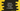# Python program to find the cube sum of first n numbers## Introduction :

This program will show you how to get the cube sum of first n natural numbers in python. The program will take the value of n as an input from the user, calculate the sum of cube and print it out.

We will solve this problem by using one loop and recursively.

## Method 1: Using a loop :

Get the value of n, call one method to find the total cube sum and use one loop to find that out :

``````def findCubeSum(n):
sum = 0
for value in range(1, n+1):
sum += value**3
return sum

n = int(input("Enter the value of n : "))

print("Cube sum : ", findCubeSum(n))``````

### Sample Output :

``````Enter the value of n : 5
Cube sum :  225

Enter the value of n : 10
Cube sum :  3025

Enter the value of n : 4
Cube sum :  100``````

## Method 2: Recursive approach :

We can also call the same method recursively to find out the cube sum :

``````def findCubeSum(n):
if(n<=1):
return 1;
return n**3 + findCubeSum(n-1)

n = int(input("Enter the value of n : "))

print("Cube sum : ", findCubeSum(n))``````

Here, findCubeSum method is called recursively. It will print the same output as the above example.